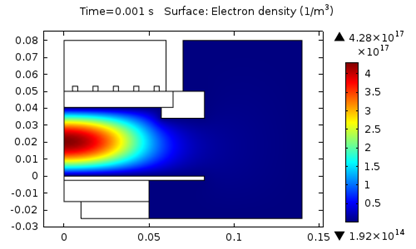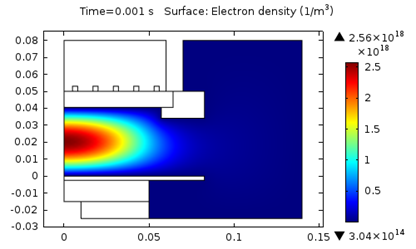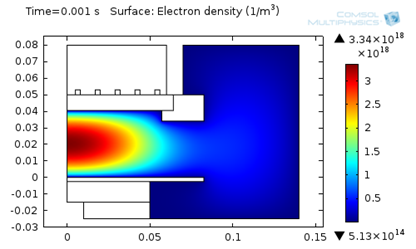# 感应耦合等离子体（ICP）中的离子温度

2014年 10月 22日

### 理论背景

\rho \frac{\partial}{\partial t}(w_k)-\nabla \cdot \mathbf{j}_k=R_k

\mathbf j_k =\rho w_k \mathbf V_k
\mathbf V_k = D_{k,m} \nabla \ln w_k + D_{k,m} \nabla \ln M-Z_k\mu_ {k,m} \mathbf E

D_{k,m}=\frac{1-w_k}{\sum_{j \ne k}^Q x_j / D_{kj}}

\mu_{k,m} = \frac{D_{k.m} q}{T_i k_B}

– \mathbf n \cdot \mathbf j_k = M_k c_k \mu_k Z_k (\mathbf n \cdot \mathbf E) \left [ ( Z_k \mathbf n \cdot \mathbf E ) > 0 \right ] + M_k R_{surf,k}

### 局部场近似 (LFA) 的使用

LFA 假定粒子的局部速度分布与局部电场平衡。因此，类似离子温度或离子迁移率等的物理量可以表示为（约化）电场中的项。LFA 要求电场的局部变化相比平均自由程的长度要小。但这并非全对，特别是在边界层中。

\mu_{Ion, red} \left [\frac1 {\text{V s cm}} \right ]= \frac{4.411\cdot 10^{19}}{\exp [0.33 \ln \{1+\exp (1.5 \ln(7.721 \cdot 10^{-3} E/n))\}]}

### 感应耦合等离子体示例

• 模型 1：设为 300 K，对应于气体温度。
• 模型 2：设为 0.1 eV (1160 K)，对应于典型的文献值。
• 模型 3：设为 D_{k,m} / \mu_{k,m}，使用 LFA 计算得到 \mu_{k,m}1. T_i = 300 \text K 4.3E17 4.1 387
2. T_i = 0.1 \text {eV} 2.6E18 2.8 407
3. 局部场近似 3.3E18 2.3 41

#### 评论 (7)

##### 留言##### 刘 阳
2016-02-26##### 彦鹏 王
2017-11-18##### 宇航 秦
2017-11-20##### 宇航 秦
2017-11-20##### 亚萌 薛
2018-01-04##### peiqi chen
2018-10-122018-10-19